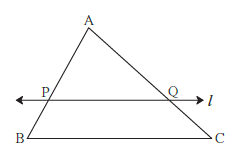# Converse of Basic Proportionality Theorem

#### notes

Theorem : If a line divides any two sides of a triangle in the same ratio, then the line is parallel to the third side.

In figure 1.18, line l interesects the side AB and side AC of D ABC in the points P and Q respectively and (AP)/(PB)
= (AQ)/(QC), hence line l || seg BC.

This theorem can be proved by indirect method.If you would like to contribute notes or other learning material, please submit them using the button below.